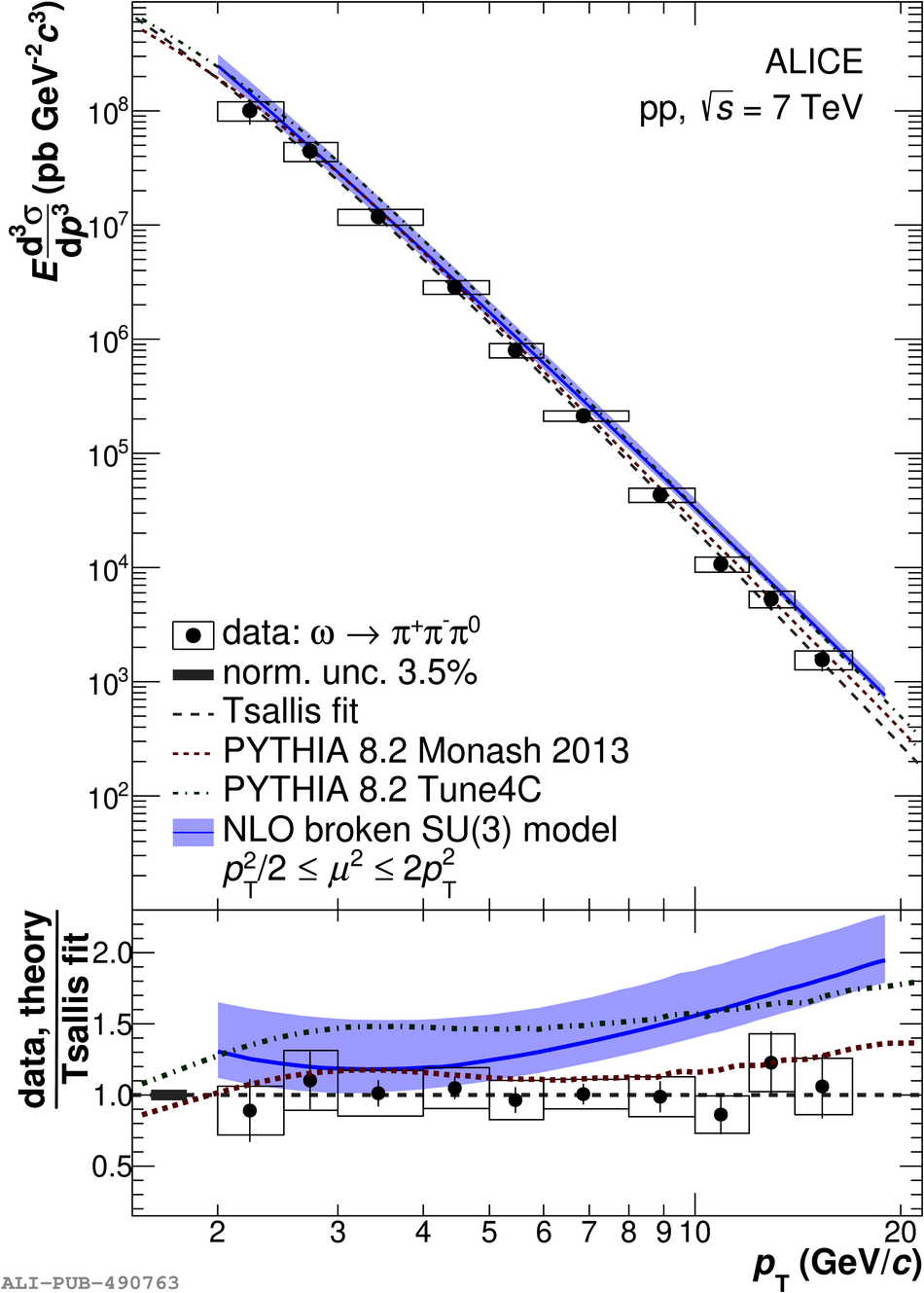# Figure 3

 Invariant cross section of $\omega$ meson production in pp collisions at $\sqrt{s}=\SI{7}{TeV}$ compared to theoretical predictions The statistical and systematic uncertainties are represented by vertical bars and boxes, respectively A Levy-Tsallis function was used to parametrise the spectrum, where the obtained fit parameters are given in \Tab{tab:FitParam} The predictions are obtained using \Pythia 8.2  with the Monash 2013  and 4C  tunes Furthermore, a NLO calculation  incorporating a model dedicated to describe vector-meson fragmentation is shown, where the band denotes the uncertainty of the scale $\mu$, which was used for factorisation, renormalisation and fragmentation In the bottom panel, the ratios of the theoretical estimates to the Levy-Tsallis fit of the measurement are shown; the ratio of the data to the Levy-Tsallis fit is also presented